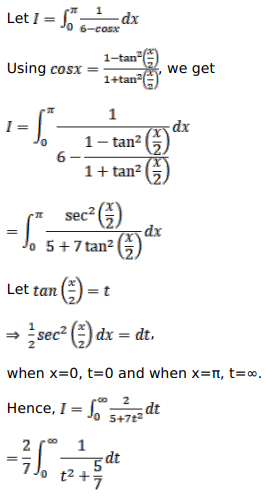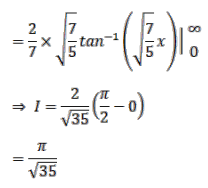# Evaluate the following integralsQuestion:

Evaluate the following integrals

$\int_{0}^{\pi} \frac{d x}{(6-\cos x)}$

Solution: